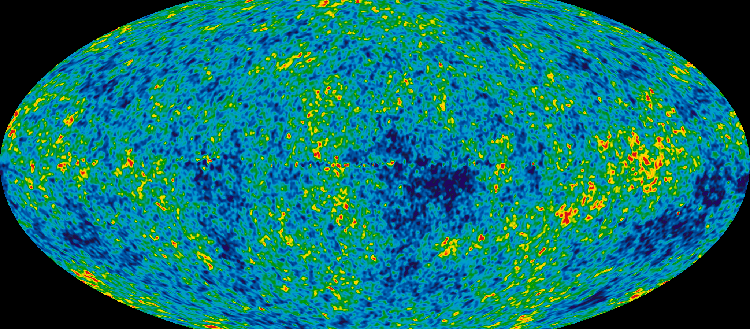# Providing a solution to the worst-ever prediction in physics

### A UNIGE physicist has proposed a new approach to solving one of the biggest theoretical problems in physics: the cosmological constant.The cosmological constant, introduced a century ago by Albert Einstein in his theory of general relativity, is a thorn in the side of physicists. The difference between the theoretical prediction of this parameter and its measurement based on astronomical observations is of the order of 10121. It’s no surprise to learn that this estimate is considered the worst in the entire history of physics. In an article to be published in Physics Letters B, a researcher from the University of Geneva (UNIGE), Switzerland, proposes an approach that may seemingly resolve this inconsistency. The original idea in the paper is to accept that another constant – Newton’s universal gravitation G, which also forms part of the equations on general relativity – may vary. This potentially major breakthrough, which has been positively received by the scientific community, still needs to be pursued in order to generate predictions that can be confirmed (or refuted) experimentally.

“My work consists of a new mathematical manipulation of the equations of general relativity that finally makes it possible to harmonise theory and observation on the cosmological constant,” begins Lucas Lombriser, assistant professor in the Department of Theoretical Physics in UNIGE’s Faculty of Sciences and sole author of the article.

Expansion in full acceleration

The cosmological constant Λ (lambda) was introduced into equations on general relativity by Einstein over a century ago. The celebrated physicist needed the constant to ensure that his theory would be compatible with a universe he believed was static. However, in 1929 another physicist – Edwin Hubble – discovered that the galaxies are all moving away from each other, a sign that the universe is actually expanding. On learning this, Einstein rued the fact that he had introduced the cosmological constant, which had become useless in his eyes, and even described it as “the greatest blunder of my life”.

In 1998, the precise analysis of distant supernovae offered proof that the expansion of the universe, far from being constant, is actually accelerating, as though a mysterious force is swelling the cosmos more and more rapidly. The cosmological constant was then once more called on in order to describe what physicists call “vacuum energy” – an energy whose nature is unknown (we talk about dark energy, quintessence, etc.) but which is responsible for the accelerated expansion of the universe.

The most precise observations of supernovae, and especially of the cosmic microwave background (microwave radiation that comes from all parts of the sky and which is considered to be left over from the Big Bang), have made it possible to measure an experimental value for this cosmological constant. The result is a very small figure (1.11 × 10 -52 m-2 ) that is nevertheless large enough to generate the desired effect of accelerated expansion.

Huge gap between theory and observation

The problem is that the theoretical value of the cosmological constant is very different. This value is obtained using quantum field theory: this holds that pairs of particles on a very small scale are created and destroyed almost instantaneously at every point of space and at any moment. The energy of this “vacuum fluctuation” – a very real phenomenon – is interpreted as a contribution to the cosmological constant. But when its value is calculated, an enormous figure is obtained (3.83 × 10+69 m-2), which is largely incompatible with the experimental value. This estimate represents the largest gap ever obtained (by a factor of 10+121, i.e. a “1” followed by 121 «0») between theory and experiment across science.

This problem of the cosmological constant is one of the “hottest” subjects in current theoretical physics, and it is mobilising numerous researchers around the world. Everyone is looking at the equations of general relativity from all sides in an attempt to unearth ideas that will solve the question. Although several strategies have been put forward, there is no general consensus for the time-being.

Professor Lombriser, for his part, had the original idea a few years ago of introducing a variation into the universal constant of gravitation G (Newton’s) which appears in Einstein’s equations. This means that the universe in which we live (with a G of 6.674 08 × 10 -11 m3 / kg s2) becomes a special case among an infinite number of different theoretical possibilities.

After numerous developments and hypotheses, professor Lombriser’s mathematical approach means it is possible to calculate the parameter ΩΛ (omega lambda), which is another way of expressing the cosmological constant but which is much easier to manipulate. This parameter designates also the current fraction of the universe that is made up of dark energy (the rest being composed of matter). The theoretical value obtained by the Geneva-based physicist is 0.704 or 70.4%. This figure is in close agreement with the best experimental estimate obtained to date, 0.685 or 68.5%, stating that this is a huge improvement over the 10+121 discrepancy.

This initial success now needs to be followed by further analyses in order to verify whether the new framework proposed by Lombriser can be used to reinterpret or clarify other mysteries of cosmology. The physicist has already been invited to present and explain his approach in scientific conferences, which reflects the interest shown by the community.

28 Aug 2019